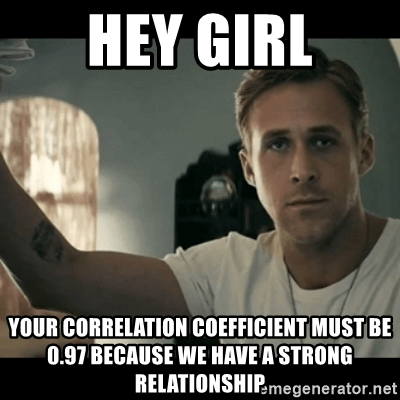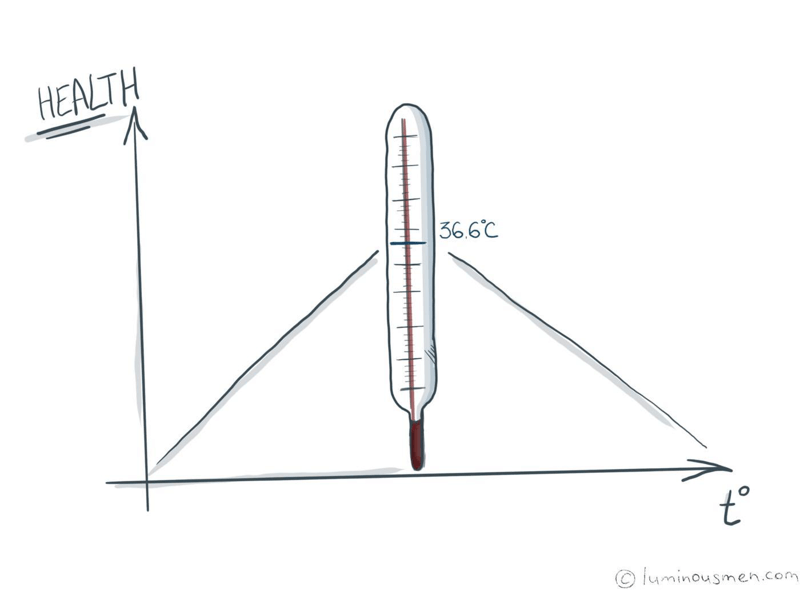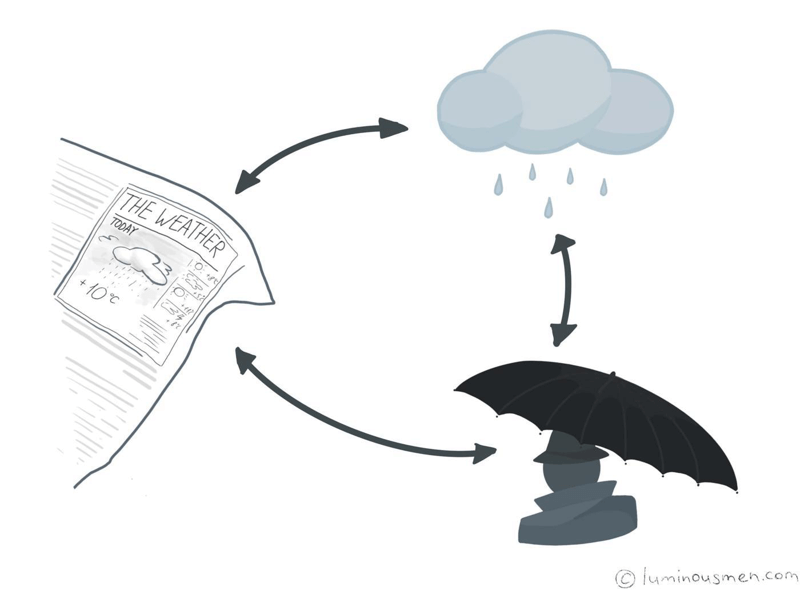There are a lot of engineers who have never been involved in statistics or data science. So, to build data science pipelines or rewrite produced by data scientists code to an adequate, easily maintained code many nuances and misunderstandings arise from the engineering side. For these Data/ML engineers and novice data scientists, I make this series of articles. I'll try to explain some basic approaches in plain English and, based on them, explain some of the Data Science model concepts.

The whole series:

Correlation, correlation dependence — the dependence or association of two or more variables. Its essence lies in the fact that when the value of one variable changes, a change (decrease or increase) of another variable occurs.

When calculating correlations, we are trying to determine whether there is a statistically significant relationship between two or more variables in one or several samples. For example, the relationship between height and weight, the relationship between performance and results of the IQ test, between work experience and productivity.

If you read somewhere a phrase like "it turned out that these events have such a correlation here" then in about 99% of cases, unless otherwise specified, we are talking about the Pearson correlation coefficient. It's the default correlation per se.

## Pearson Correlation CoefficientSuppose we have two processes, for each of which we measure some parameters. As a result, we have a set of pairs of numbers(value of the 1st process and value of the 2nd process). Assuming at the same time these processes are somehow connected, we assume that this connection should reveal itself numerically from the parameters' values. Hence, from the resulting pairs of numbers, we can somehow get information about the presence or absence of a connection.

However, interconnection can be of varying degrees of strength; therefore, it is desirable to obtain not a binary "yes" or "no", but something like a continuous number which will characterize the magnitude of the degree (strength) of the interconnection between two variables. And here, meet, the Pearson correlation coefficient.

It can vary from -1 (negative correlation) to +1 (positive correlation). If the correlation coefficient is 0 then this indicates the absence of interconnection between the variables. And if the correlation coefficient is closer to 1 (or -1), then it is a strong correlation, and if closer to 0, a weak correlation.

With a positive correlation, an increase (or decrease) in the values of one variable leads to a regular increase (or decrease) in another variable. With a negative correlation, an increase (or decrease) in the values of one variable leads to a regular decrease (or increase) in another variable.

The formula for Pearson correlation coefficient for two variables X and Y:

$$r=\frac{cov}{\sigma_x\sigma_y}$$

where $$cov=M((X - M(X))(Y - M(Y))$$

Frankly speaking, I also do not like the mathematical version of the coefficient. Some scary little letters in scary combinations, all that(I get you guys). Plain text with examples seems much more understandable. Therefore, I will try to follow him.

There is such a term "mathematical expectation of a quantity", for brevity called expectation or mean(we talked about it in the previous post). In the simplest case, its meaning is extremely simple: it is the arithmetic average of all values:

$$M(X)=\frac{(x_1 + x_2 + ... + x_n)}{n}$$

Next, we find for each number in the list its deviation from the mean:

$$mx = M(X)$$

$${dx_1, dx_2, ...} = {x_1 - mx, x_2 - mx, x_3 - mx, ...}$$

The same can be done with another list in which there are measurements of the second variable, measured simultaneously with the first:

$$Y={y_1, y_2, ..., y_n}$$

$$my = M(Y)$$

$${dy_1, dy_2, ...} = {y_1 - my, y_2 - my, ..., y_n - my}$$

For example, we measured the temperature of every patient in the ward. And, besides that, we recorded how many aspirin pills the patient took every day. By the procedure above we can build the list of deviations of every patient in the ward from the mean temperature of the patients. And apply the same procedure to the corresponding list of deviations of the number of aspirin pills taken by the patient from the average number of pills taken.

Hence, we can assume that if temperature somehow is connected with a number of aspirin pills taken then the deviations will be connected with them as well.

For example, if taking aspirin leads to an increase in temperature, then we will see the following. If the patient took more pills than others, then his temperature deviates from the average upwards. If the patient took fewer pills than others, then his temperature deviates from the average downwards. That is both deviations — in the same direction.

If we multiply in pairs all the deviations, then the result will be positive.

And vice versa — if taking aspirin lowers the temperature, then all products of deviations will be negative.

In other words, we have obtained a certain indicator with an interesting property — it is positive for the positive relation of phenomena and negative for negative relation.

$$D={{dx_1 dy_1,dx_2 dy_2,...}}$$

But after all, if variables are not connected, then on a large number of measurements approximately both of them should be equally distributed(we will cover this topic in the next post) — a positive product of deviations and a negative one. If you add them up, then, apparently, you get something around zero. Moreover, the closer to zero, the more measurements were there.

Yeah, it mean again. Therefore, here it is, the criteria — covariance:

$$cov = M(D)$$

The covariance can have an arbitrary value, and therefore, to conclude the relationship between the lists, it is also necessary to know the maximum range just for these variables.

To do this, we introduce another interesting value — derived from the list of squares of deviations from the mean.

$$DX^2 = {dx_1^2, dx_2^2, ...}$$

$$\sigma_x = \sqrt{M(DX^2)}$$

It is called "standard deviation".

So, it can be shown that the covariance in its absolute value does not exceed the product of the standard deviations of these two lists.

$$|cov| ≤ \sigma_x * \sigma_y$$

Well, since the standard deviation by construction is always positive, we can conclude that

$$-\sigma_x*\sigma_y ≤ cov ≤ \sigma_x*\sigma_y$$

or

$$r=\frac{cov}{\sigma_x\sigma_y}$$

$$-1≤r≤1$$

In general, if we take such coefficient as a measure of association, then it will turn out to be very convenient and very universal: it will show whether the values are related to each other and how much.

Everything is very convenient and universal, but in the above reasoning, there is a fair amount of flaws, which, given the universality of the obtained "measure of association", is very convenient to ignore but they can lead to wrong conclusions.

### Example #1

There once was a student who decided to find out the connection between the patient's body temperature and the patient's well-being. Everything is obvious, at a normal temperature of 36.6 ° C, the state of health seems to be the best. When the temperature rises, health deteriorates. However, when the temperature drops, health also deteriorates...If the measures on the left and right of the normal temperature are symmetrical(see on the picture), the correlation will be close to zero. From which the student concluded that there is no connection between temperature and well-being(ha!).

### Example #1

The example shows: the "measure of association" introduced by us is not universal. The reasoning made at its construction applies only to linear dependencies.

Roughly speaking, this only works when an observed dependency is the following:

$$y(x) = kx$$

If the dependency is different, then, generally speaking, the correlation coefficient may be random. Yes, yes, not zero, but random.

In other words, a researcher somehow must know in advance that there is either a linear relationship or there is no association at all to make more or less correct conclusions.

But how can he know this before research?

Only from other studies. Where the analysis was done by other methods. For example, it can be based on a thoughtful examination of graphs or distributions.

But if all this has already been done, then why should he even consider the correlation at all? He already has more reliable results.

How good is such a measure, which gives a random number, and thus leads to completely different conclusions?

As a result, it turns out that the only thing that can be done with the help of correlation is the conclusion that there is no linear relationship throughout the interval. It is all about the absence — even the existence of such a relationship cannot be concluded.

And the same thing will be with aspirin pills that have an optimal dose — with this dose, the results will be the best, but with a smaller and with a larger one, they will be worse.

### Example #2

There once a scientist. Each time in the morning he saw the same person passing by his window. After some time, he noticed an amazing pattern — if this person took an umbrella with him, then it was raining that day.

Of course, this did not happen every time — sometimes the person took an umbrella, but it did not rain, sometimes he did not take it, but it still rained. But too often the presence of an umbrella with rain and the absence of an umbrella with no rain occurred at the same time.

The scientist, of course, did not trust such his hypothesis, but instead began to carefully write down his observations every day. After a while, he attributed the events "an umbrella" and "rain" as 1, the events "no umbrella" and "no rain" as 0, and counted the correlation according to the records.

The correlation was very high: 0.95. These two events were linked!

A proud scientist wrote an article entitled "How to cause rain" in which he convincingly proved that it was this guy who controls the rain at his place of residence by wearing an umbrella.

After all, such a high correlation can not be the result of chance.

### Example #2If the correlation coefficient showed a real connection of one variable with another (and it is not a random number, as it was with a student), it still does not prove that one phenomenon was caused by another. It may be that the first phenomenon is not caused by the second, and even the second phenomenon is not caused by the first.

In these cases, they say — "it may be that there is a certain third phenomenon that causes both two" — but in some cases, this is more misleading than helpful. After all, the truth may be such a phenomenon, but all the same, the possible options do not end there.

And, moreover, no one guarantees that it is the "third phenomenon" that someone suggested is the causation of those two.

Of course, there is no external mystical power in the story above. The umbrella guy simply watched the weather forecast in the morning, and, if it promised rain, he would take an umbrella with him.

It may seem that, well, if not mystical power, but still the third phenomenon that causes the first two, is there.

Suppose there is. But which one?

Does the weather forecast causes the rain?

Or has the future rain causes the forecast?

Well okay, not the rain itself, but the atmospheric pressure, the speed, and direction of the wind, the presence of the lake, etc., and cause rain and forecast?

However, this person gets an umbrella in the mornings because he simply reads the published forecasts and doesn't know the upcoming weather at all. To check this, we could simply ask the forecast source in which he reads these forecasts to publish incorrect forecasts. And thus, to see firsthand that the umbrella is taken if it is written in the forecast that it will rain, and not at all if some physical parameters indicate its high probability.

That is, there is no one "third phenomenon" that causes the first two. Instead, there is a whole set of phenomena that are in very difficult relationships with each other.

## Conclusion

The moral of the stories is that no statistical indicator alone can confirm the theory you like. Theories are confirmed only by a set of indicators of a correctly constructed series of experiments. A series, not a single experiment — even with a large number of data. We will talk

Whatever the statistics you have calculated, it only gives you some ground for hypothesis and assumptions(take a look at how Nicolas cage causes people to die in the pool). For hypotheses, and not for "theories", which many people like to declare at the first stage.

The first experiment is the first. According to its results, you need to formulate a hypothesis and in the following experiments check whether it gives true predictions.

The intention in the first experiment is the data on which the hypothesis is built. It is impossible to check on these data whether the hypothesis works: it is on them you built it - the stump is clear, it will work on them. So it will be with any hypothesis - even with the wrong one.

With the predictions that have come true on the new experiments, the truth will already appear "statistical evidence" — after all, the proposed dependence of one phenomenon on another allows you to make predictions on the data that we had not yet received as a hypothesis at the time of its introduction. This proves the reality of the connection and not just a high correlation.

Moreover, it is not enough to repeat the same experiment and make sure that it worked a second time. It worked or not, but you have to check all this and in other conditions, too. After all, a real theory cannot describe one particular case — it should extend to a rather extensive area of ​​possible options.

But this is not the end of it — even if this hypothesis does provide predictions, it is still necessary to check the following experiments that all alternative hypotheses do not work for them. Otherwise, it turns out that you did not prove the correctness of your hypothesis, but only the correctness of a rather extensive set of hypotheses, including yours.

Such is the science of data.

Support author